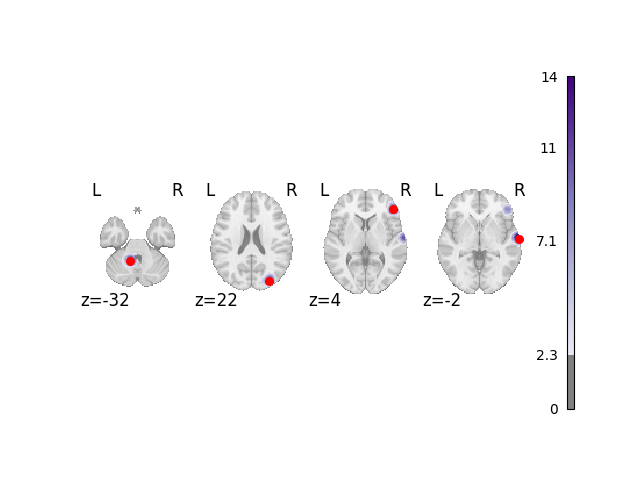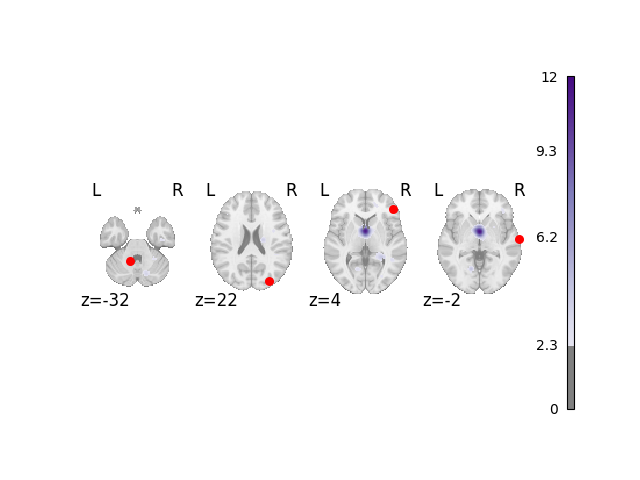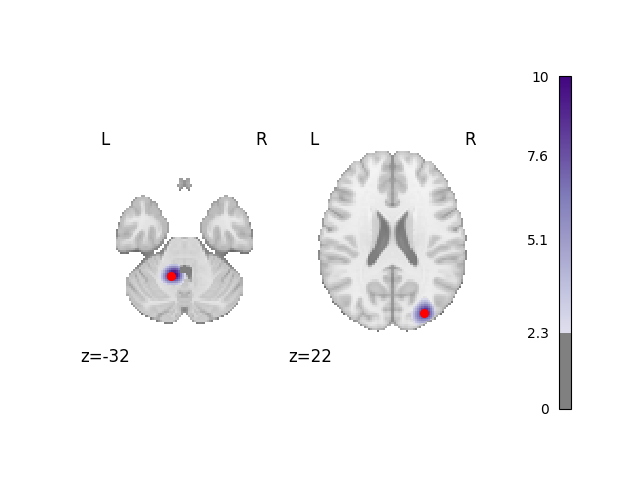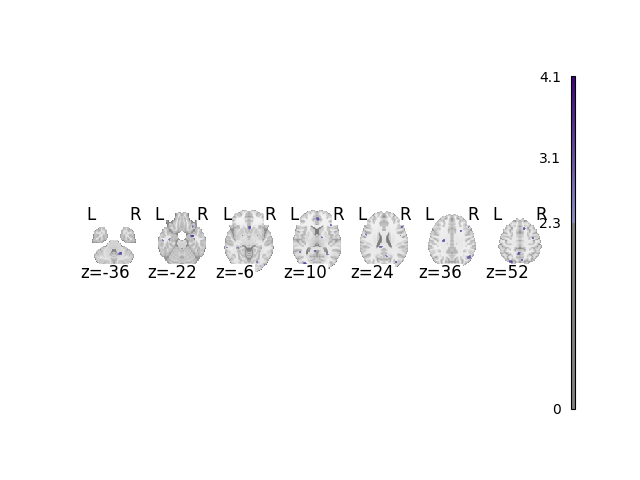# Simulate data for coordinate based meta-analysis

Simulating data before you run your meta-analysis is a great way to test your assumptions and see how the meta-analysis would perform with simplified data

```import matplotlib.pyplot as plt
from nilearn.plotting import plot_stat_map

from nimare.correct import FDRCorrector
from nimare.generate import create_coordinate_dataset
from nimare.meta import ALE
```

## Create function to perform a meta-analysis and plot results

```def analyze_and_plot(dset, ground_truth_foci=None, correct=True, return_cres=False):
meta = ALE(kernel__fwhm=10)
results = meta.fit(dset)
if correct:
corr = FDRCorrector()
cres = corr.transform(results)
else:
cres = results

# get the z coordinates
if ground_truth_foci:
stat_map_kwargs = {"cut_coords": [c for c in ground_truth_foci]}
else:
stat_map_kwargs = {}

fig, ax = plt.subplots()
display = plot_stat_map(
cres.get_map("z"),
display_mode="z",
draw_cross=False,
cmap="Purples",
threshold=2.3,
symmetric_cbar=False,
figure=fig,
axes=ax,
**stat_map_kwargs,
)

if ground_truth_foci:
# place red dots indicating the ground truth foci

if return_cres:
return fig, cres

return fig
```

## Create Dataset

In this example, each of the 30 generated fake studies select 4 coordinates from a probability map representing the probability that particular coordinate will be chosen. There are 4 “hot” spots centered on 3D gaussian distributions, meaning each study will likely select 4 foci that are close to those hot spots, but there is still random jittering. Each study has a `sample_size` sampled from a uniform distribution from 20 to 40. so some studies may have fewer than 30 participants and some more.

```ground_truth_foci, dset = create_coordinate_dataset(foci=4, sample_size=(20, 40), n_studies=30)
```

## Analyze and plot simple dataset

The red dots in this plot and subsequent plots represent the simulated ground truth foci, and the clouds represent the statistical maps of the simulated data.

```analyze_and_plot(dset, ground_truth_foci)
```Out:

```<Figure size 640x480 with 6 Axes>
```

## Fine-tune dataset creation

Perhaps you want more control over the studies being generated. you can set:

• the specific peak coordinates (i.e., `foci`)

• the percentage of studies that contain the foci of interest (`foci_percentage`)

• how tightly the study specific foci are selected around the ground truth (i.e., `fwhm`)

• the sample size for each study (i.e., `sample_size`)

• the number of noise foci in each study (i.e., `n_noise_foci`)

• the number of studies (i.e., `n_studies`)

```foci = [(0, 0, 0)]
foci_percentage = 1.0
fwhm = 10.0
n_studies = 30
sample_sizes =  * n_studies
sample_sizes = 300
n_noise_foci = 10

_, manual_dset = create_coordinate_dataset(
foci=foci, fwhm=fwhm, sample_size=sample_sizes, n_studies=n_studies, n_noise_foci=n_noise_foci
)
```

## Analyze and plot manual dataset

```fig = analyze_and_plot(manual_dset, ground_truth_foci)
fig.show()
```## Control percentage of studies with the foci of interest

Often times a converging peak is not found in all studies within the meta-analysis, but only a portion. We can select a percentage of studies where a coordinate is selected around the ground truth foci.

```_, perc_foci_dset = create_coordinate_dataset(
foci=ground_truth_foci[0:2], foci_percentage="50%", fwhm=10.0, sample_size=30, n_studies=30
)
```

## Analyze and plot the 50% foci dataset

```fig = analyze_and_plot(perc_foci_dset, ground_truth_foci[0:2])
fig.show()
```## Create a null dataset

Perhaps you are interested in the number of false positives your favorite meta-analysis algorithm typically gives. At an alpha of 0.05 we would expect no more than 5% of results to be false positives. To test this, we can create a dataset with no foci that converge, but have many distributed foci.

```_, no_foci_dset = create_coordinate_dataset(
foci=0, sample_size=(20, 30), n_studies=30, n_noise_foci=100
)
```

## Analyze and plot no foci dataset

When not performing a multiple comparisons correction, there is a false positive rate of approximately 5%.

```fig, cres = analyze_and_plot(no_foci_dset, correct=False, return_cres=True)
fig.show()

p_values = cres.get_map("p", return_type="array")
# what percentage of voxels are not significant?
non_significant_percent = ((p_values > 0.05).sum() / p_values.size) * 100
print(f"{non_significant_percent}% of voxels are not significant")
```Out:

```94.07702104751776% of voxels are not significant
```

Total running time of the script: ( 0 minutes 18.011 seconds)

Gallery generated by Sphinx-Gallery